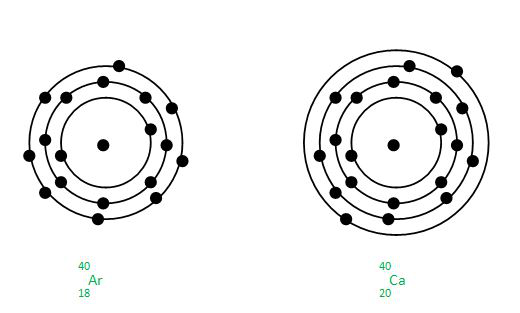# Difference between Isobars and Isotopes

• Last Updated : 30 Nov, 2021

A chemical element’s atoms are always made up of a nucleus surrounded by an electron cloud. Protons and neutrons make up the nucleus. The number of protons in each atom of a given element is always the same. This indicates that a chemical element’s number of protons is a one-of-a-kind attribute. However, the number of neutrons in the nucleus of atoms of the same element might differ.

Isotopes are the names for these various types. The sum of protons and neutrons in an element’s nucleus is the atomic mass of that element. Even if their atomic numbers are different, the atomic masses of various elements can sometimes be the same. Isobars are the names given to these various types.

The significant distinction between isotopes and isobars is that isotopes have different atomic masses from one other, whereas isobars have comparable atomic masses.

### Isotopes

It has been found in many experimental types of research that there are some elements whose atoms have the same atomic number that of the element but mass numbers are different such atoms of elements are called the isotopes of that element.

Isotopes can be considered as variants of the same element, the only difference is in their number of protons and number of neutrons which exists in the nuclei of atoms. The number of neutrons and the number of protons together makes the mass number of an element so the mass number of isotopes is different.

Let’s understand by the example of isotopes of Carbon, Carbon has 12C, 13C and 14C as its isotopes mean 12, 13 and 14 are the mass number of Carbon and atomic number is 6. But as atomic number means the number of protons so all carbon isotopes will have the same number of atoms while the number of neutrons is different.

The credit to finding isotopes goes to the British physicist J.J. Thomson who was the person to discover isotopes of the Neon element which has atomic number 10 and it is actually a gas. Some isotopes are found to be radioactive means they have a radioactive decay effect so the nuclei of radioactive isotopes will be unstable. An example of radioactive isotopes is uranium which has two isotopes with mass numbers 235 and 238.

Applications of Isotopes:

• Goitre (swelling neck) disease can be cured by using isotope of iodine.
• Treatment of some type of cancer can be done using cobalt isotope.
• Uranium isotope is used as nuclear fuel.

Isobars:

Suppose there are two or more elements which have different atomic number but their mass number is same then these elements are known as Isobars. Isobars are actually different elements with different number of protons and neutrons but sum of number of protons and neutrons are same for these different elements and hence they show similar physical properties. The credit to find isobars goes to Alfred Walter Stewart who discovered isobars in 1918. Some isobars are also radioactive in nature.  For radioactivity of isobars there is a rule known as Mattauch Isobar Rule. According to this rule if two adjacent elements on the periodic table have isotopes of the same mass number, then at least one of these isobars must be radioactive

Example:

Argon and Calcium are Isobars as both have same mass number and atomic number is different.

Applications of Isobars:

• Isobars are used in medical field to treat tumours and blood clots.
• Thyroid disorders can be treated using isobars of iodine.
• Blood cancer can be treated using isobar of phosphorus.

Difference between Isotopes and Isobars :

### Sample Questions

Question 1: Chemical properties of isobars are same or different explain?

Chemical properties of any element depends mainly on number of electrons and isobars have different number of electrons as atomic number is different so chemical properties of isobars are different.

Question 2: Physical properties of isobars are same or different explain?

Physical properties are mainly governed by number of nucleons or say atomic mass, as atomic mass of isobars are equal hence isobars has same physical properties.

Question 3: Which mass should be taken in case of isotopes as they have different atomic masses?

It is clear that isotopes have same atomic number but different mass number so for calculation purposes concept of average atomic mass is taken. Lets say there are three isotopes of some element X then for practical purposes first calculate the average atomic mass of the isotopes like (m1+m2+m3)/3 if they exist in nature in same ratio.

Question 4: Calculate average atomic mass of chlorine isotopes which occur in nature in 3:1 and has masses of 35 u and 37 u?

Let’s take 3:1 which means if we have 100 units chlorine then 75 units of chlorine is of 35 u mass and remaining 25 units will have a mass of 37 u.

Now calculating average atomic mass of chlorine

=  [35×(75/100) + 37×(25/100)]

= [(105/4)+(37/4)]

= 142/4

= 35.5 u

Question 5: Give the electronic configuration of Argon and Calcium isobar.